Your browser doesn't support the features required by impress.js, so you are presented with a simplified version of this presentation.

For the best experience please use the latest Chrome, Safari or Firefox browser.# toadaptive physical and bio inspireddata driven approaches

Gastone Pietro Rosati Papini
http://tonegas.it - gastone.rosatipapini@unitn.it# The robotics challenges

• Dealing with humans
• Dealing with uncertainties environment
• Guarantee safety
• Tradeoff between safety e efficiency
• Objective generalization out from design phase
• Dealing with failures
• Dealing with partial and uncertain measure of the environment
• Dealing with deformable objects (difficult to model)

Are standard model-based approaches sufficient?

— DARPA challenge, 2015.

# Model based approaches ⇄

Limits:
• Difficult to model everything
• Deal with un-modeled situations
• Mechanical systems are known
• Behaviour is predictable
• Stability and safety guaranteed

# Model based approaches ⇄ Data driven approaches

Limits:
• Difficult to model everything
• Deal with un-modeled situations
• Mechanical systems are known
• Behaviour is predictable
• Stability and safety guarantees
Limits:
• Black-box structure, poor safety
• Huge amount of data
• Time to collect and label data
• Dangerous to collect data
• Flexible
• Modular
How can the two approaches be combined?

# Model based approaches ⇄ Data driven approaches

Limits:
• Difficult to model everything
• Deal with un-modeled situations
• Mechanical systems are known
• Behaviour is predictable
• Stability and safety guarantees
Limits:
• Black-box structure, poor safety
• Huge amount of data
• Time to collect and label data
• Dangerous to collect data
• Flexible
• Modular
Using physical and biological inspiration to structure the neural network and guide the process of learning

# From my background to the future research project

University and the Master Thesis at the University of Pisa

# University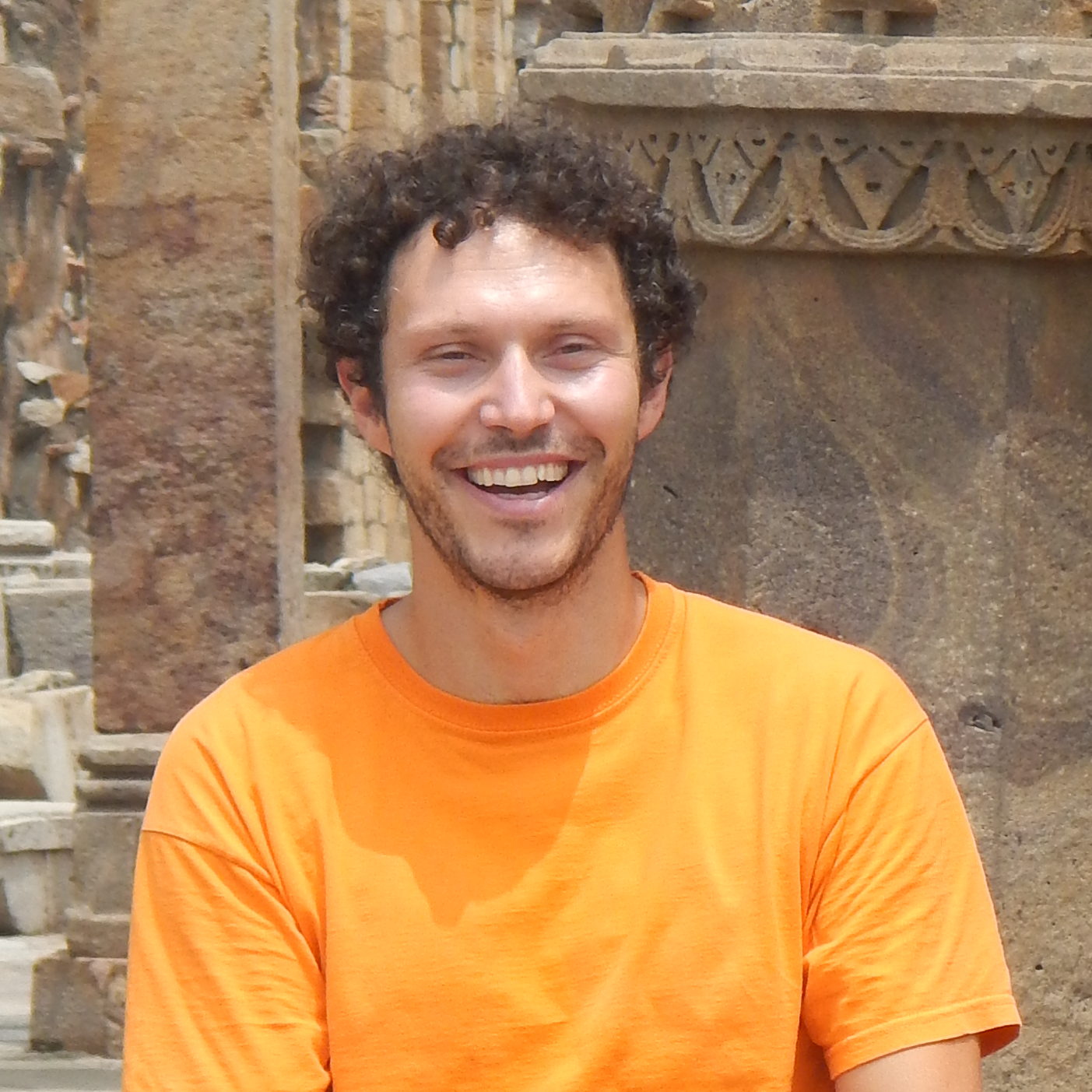Bachelor's Degree

Software Engineering
• Programming languages
• Software skill
• Realtime systems
• Networking devices
Master's Degree

Automation engineering
• Mechatronics system
• Mechanic modelling
• Control theory
• Robotics:(){ :|:& };:
University

# Master Thesis - Robust admittance control for Body Extender

Carlo Alberto Avizzano - Advisor
Antonio Bicchi - Co-Advisor

Rosati Papini, G.P. Master Thesis "Controllo robusto di forza per una struttura robotica articolata di tipo Body Extender", 2012.
Rosati Papini, G.P. and Avizzano, C.A. "Transparent force control for Body Extender." 2012 IEEE RO-MAN.
Master Thesis

# Control System - on single joint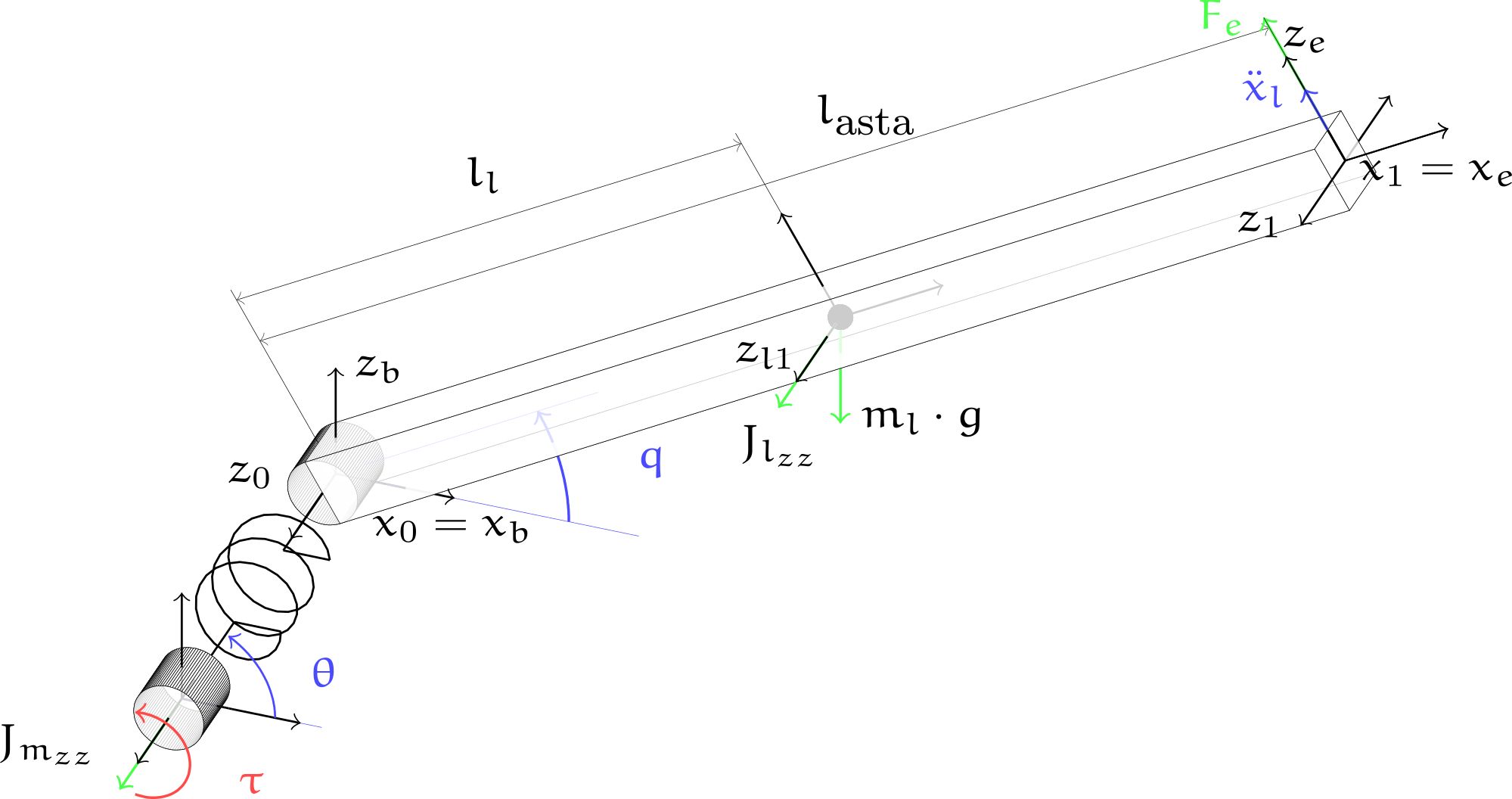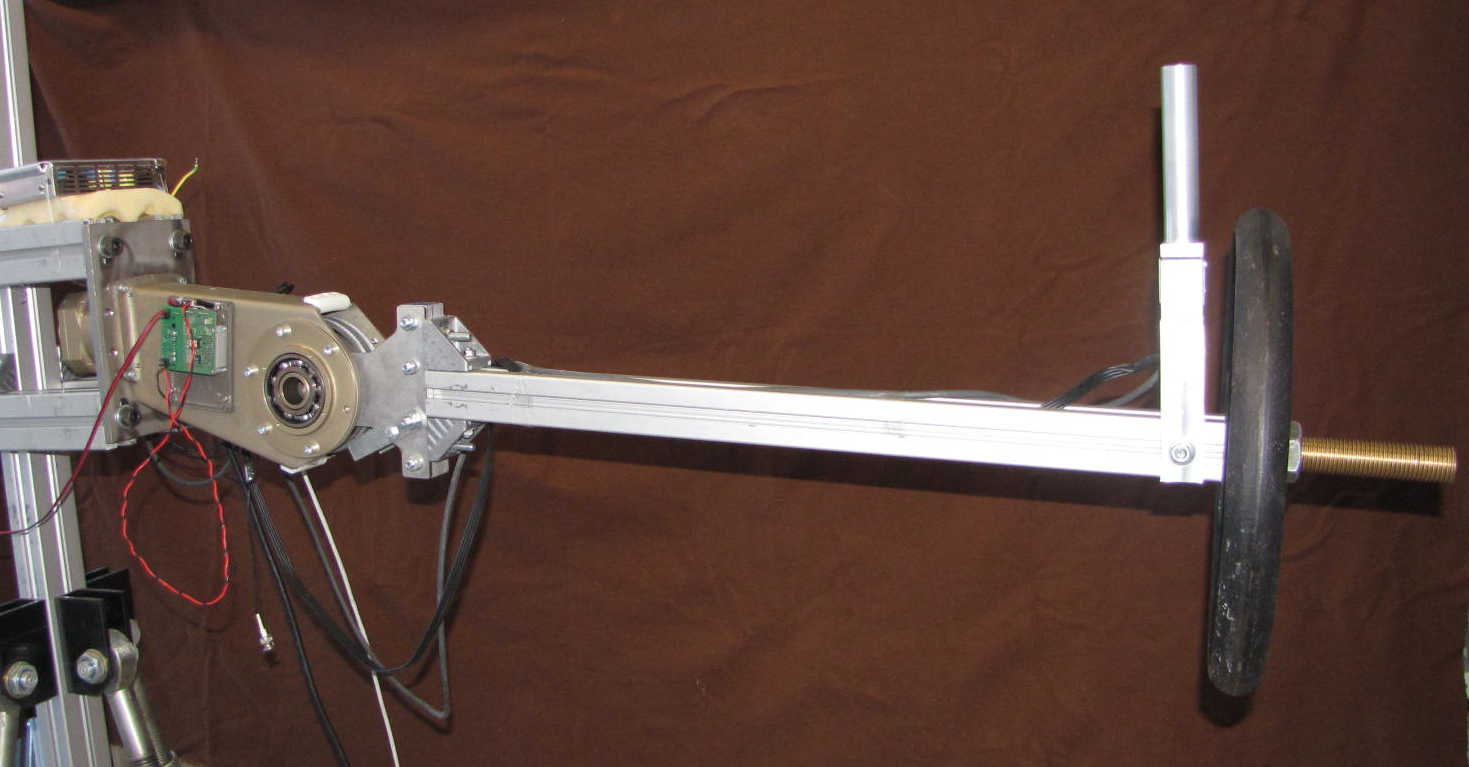Control System

# Parameter Estimator

Why is needed?
• Because the environment is changing continuously
Simple solution →Least square estimator
$$Y_{e}=\left[\begin{array}{c} {Y\left(q, \dot{q}_{r}, \ddot{q}_{r}\right)} \\ {Y\left(q, \dot{q}_{r}, \ddot{q}_{r}\right)} \\ {\vdots} \\ {Y\left(q[n], \dot{q}_{r}[n], \ddot{q}_{r}[n]\right)} \end{array}\right] \quad \Gamma=\left[\begin{array}{c} {\tau+J^{\top}(q) F_{s}} \\ {\tau+J^{\top}(q) F_{s}} \\ {\vdots} \\ {\tau[n]+J^{\top}(q[n]) F_{s}[n]} \end{array}\right]$$
$$\pi^{\top} =\left(Y_{e}^{\top} Y_{e}\right)^{-1} Y_{e}^{\top} \Gamma$$
• Linear regessor for parameters: $$Y\left(q, \dot{q}_{r}, \ddot{q}_{r}\right)$$
• Inertial parameters: $$\pi = \left[m_{a}, I_{a_{x}}, I_{a_{y}}, I_{a_{z}}, I_{a_{x y}}, I_{a_{x z}}, I_{a_{y z}}\right]$$
• Approx true $$q$$: $$\dot{q}_{r}=\dot{q}_{d}+\Lambda\left(q_{d}-q\right)$$
• Force sensor: $$F_s$$
Feedback Linearization
Force Control

— Siciliano, B. et al. Robotics: modelling, planning and control. Springer Science & Business Media, 2010.

# Results - Testing of the system# Model based approach - Explicit self tuning control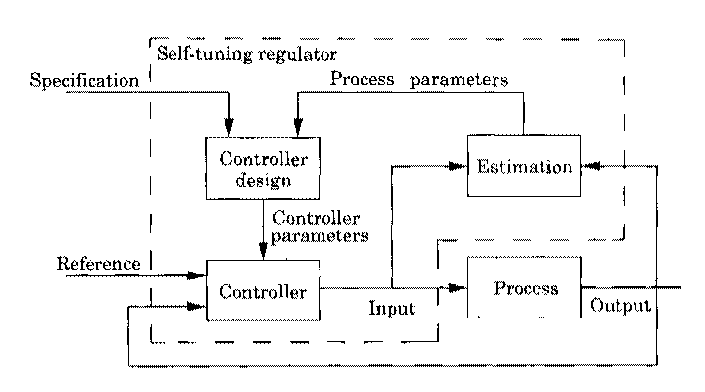The control system is an adaptive controller defined as:
• explicit self tuning control
The regulator is updated through the system parameters.

In our case the characteristics are:
• The controller is model based
• Parameter estimator (estimation) is model based

— Åström, Karl J. and Wittenmark, B. Adaptive control. Courier Corporation, 2013.

# From my background to the future research project

PhD research at Scuola Superiore Sant'Anna

# PhD - Main Activities

• Veritas (FP7-ICT 247765) - European project focused on empathic design
A desktop haptic device is employed to induce a programmable hand-tremor on healthy subjects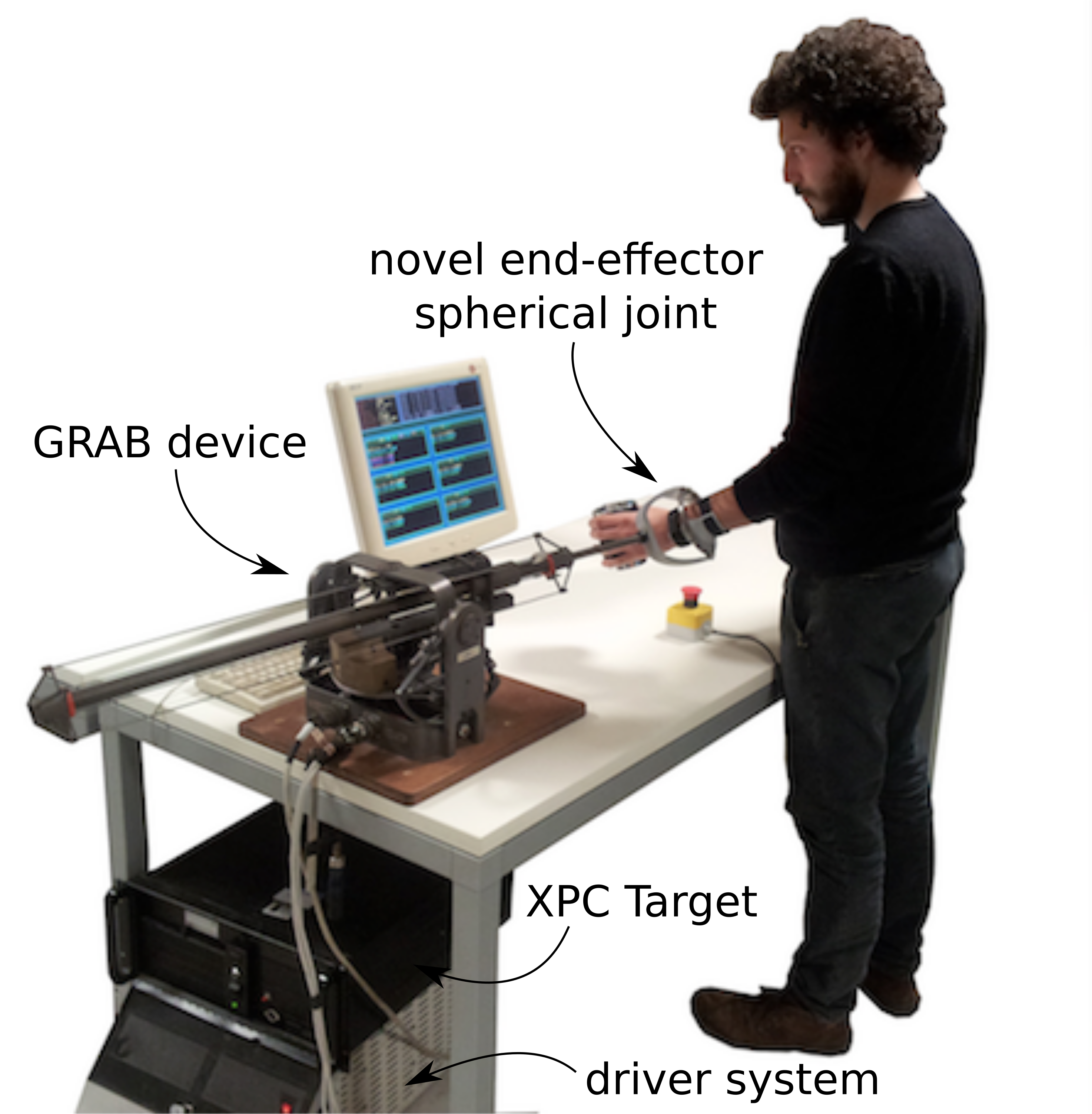PhD

# PhD - Main Activities

• Veritas (FP7-ICT 247765) - European project focused on empathic design
A desktop haptic device is employed to induce a programmable hand-tremor on healthy subjects
• PolyWec (FP7-ENERGY 309139) -
European project focused
on wave energy
and electroactive polymers
Models, control systems, simulations,
experimental tests
PhD

# Veritas Project - Desktop haptic interface for hand tremor induction

Parkinsonian user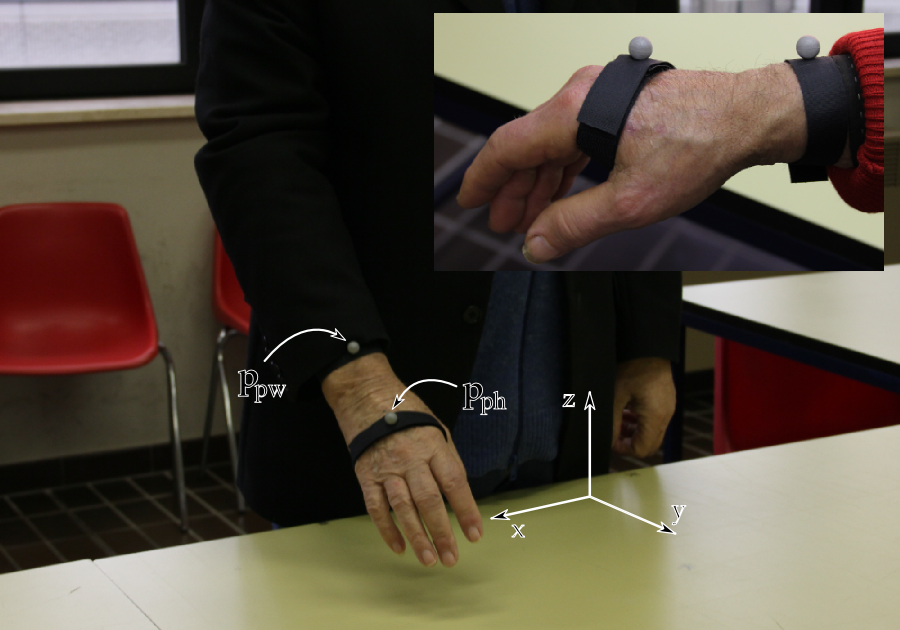Rosati Papini, G.P. et al."Haptic hand-tremor simulation for enhancing empathy with disabled users." 2013 IEEE RO-MAN.
Rosati Papini, G.P. et al."Desktop haptic interface for simulation of hand-tremor." IEEE Transactions on Haptics, 2015.Veritas

# Human impedance estimator

Why is needed?
• Because the system is used by different person• RMS amplitude with time window of 1 sec: $$E(s)$$
• Filtered wrist reference position: $$x_{pw}^*$$
• Filtered wrist position: $$\widehat{x}_{uw}^*$$
• Human impedance estimator is a PID regulator: $$M_e(s)$$
• Estimated human impedance: $$\hat{m}_{h}$$
System compensator
Position estimator

# Results - Testing of the device

Designer of Indesit company testing our device on a gas hobRosati Papini, G.P. et al."Desktop haptic interface for simulation of hand-tremor." IEEE Transactions on Haptics, 2015.

# Model based approach - Model reference adaptive controlThe control system is an adaptive controller defined as:
• Model reference adaptive systems
The adjustment mechanism set the controller parameters in such a way that the error between $$y$$ and $$y_m$$ is small.

In our case the characteristics are:
• the controller is model based
• reference amplitude estimator (model reference) is not based on physical model
• mass estimator (adjustment mechanism) is not based on physical model

— Åström, Karl J. and Wittenmark, B. Adaptive control. Courier Corporation, 2013.

# PolyWec Project - Exploiting electroactive polymers for wave energy conversion• Preliminary studies on the energy production of Poly-OWC
Compiled simulink schema for the energy production evaluation
• Hardware in the loop tests
Testing different control schemas and harvesting cycles
• Experimental tests
Implementation of control schema and video analisys for energy harvesting evaluation
• Optimal control for Poly-OWC
Real-time controller for maximizing the energy estration of the Poly-OWC

— Vertechy, R., Rosati Papini, G.P. and Fontana, M. "Reduced model and application of inflating circular diaphragm DEGs for wave energy harvesting." Journal of Vibration and Acoustic, 2015.

— Moretti, G., Rosati Papini, G.P. et al."Resonant wave energy harvester based on DEG." Smart Materials and Structures, 2018.
— Moretti, G., Rosati Papini, G.P. et al. "Modelling and testing of a wave energy converter based on DEG." Proceedings of the Royal Society A, 2019.
Rosati Papini, G.P. at al. "Experimental testing of DEGs for wave energy converters." 2015 European Wave and Tidal Energy Conference.

Rosati Papini, G.P. PhD Thesis "Dynamic modelling and control of DEG for OWC wave energy converter", 2016
Rosati Papini, G.P. et al. "Control of an OWC wave energy converter based on DEG." Nonlinear Dynamics, 2018.
PolyWEC

# Control of an OWC wave energy converter based on DEGOptimal Poly-OWC control

# Optimization Procedure

Objective
\begin{aligned} \max E_{a} &=\max \left(-\int_{0}^{T} P_{\mathrm{PTO}}(t) \mathrm{d} t\right) \\ &\approx\min _{f_{\text {PTO}}} T_{s} \sum_{k=0}^{N-1} f_{\text{PTO}}[k] \dot{\eta}[k] = \min _{f_{\text {PTO}}} T_{s} \underline{f^\top_{\text{PTO}}}\underline{\dot{\eta}}\end{aligned}
Cummin’s Equation
\begin{aligned} m_{\infty} \ddot{\eta}(t) &=-\rho_w g S \eta(t)-C_{r} x_{r}(t)+f_{e}(t)+f_{\mathrm{PTO}}(t) \\ \dot{x}_{r}(t) &=A_{r} x_{r}(t)+B_{r} \dot{\eta}(t) \end{aligned}
State Space $$\rightarrow$$ Discretization
$$\underline{\dot{\eta}}=\Omega_{V}\left(\underline{f_{\mathrm{PTO}}}+ \underline{f_{e}}\right)\;\;\;\underline{\eta}=\Omega_{P}\left(\underline{f_{\mathrm{PTO}}}+ \underline{f_{e}}\right)$$
Contraints
$$f_\mathrm{MIN}(\eta) \leq \underline{f_{\mathrm{PTO}}} \leq f _\mathrm{MAX}(\eta),\;\;\; \eta_\mathrm{MIN} \leq \underline{\eta} \leq \eta_\mathrm{MAX}$$
• extracted energy: $$E_a$$
• time window optimisation: $$T$$
• discretization time: $$T_s$$
• PTO instant power: $$P_{\mathrm{PTO}}$$
• water level: $$\eta$$
• PTO force: $$f_{\mathrm{PTO}}$$
• radiation state space: $$x_r,A_r,B_r,C_r$$
• density: $$\rho_w$$
• infinite frequency added mass: $$m_{\infty}$$
• exitation force: $$f_e(t)$$
• water surface: $$S$$
• evolution of state by input: $$\Omega_{V},\Omega_{P}$$
Optimization Procedure

# Results - Optimal CyclesRosati Papini, G.P. et al. "Control of an OWC wave energy converter based on DEG." Nonlinear Dynamics, 2018.
Optimal Cycles

# From optimal control to a real-time controller for Poly-OWC• From direct inspection of the results of the optimal control is obtained real-time controller
• Lookup table defines the optimal time to charge using $$\dot{p}_{th}$$ based on $$H_s,T_e$$

# Model based approach - Gain scheduling controller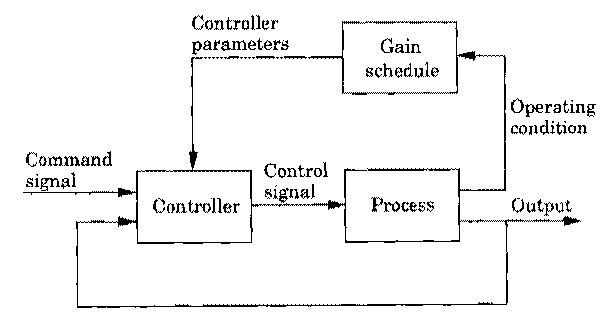The control system is an adaptive controller defined as:
• gain scheduling controller
the gain schedule adjusts the controller parameters on the basis of the operating conditions.

In out case the characteristics are:
• the control logic is derived from optimal control solutions
• the lookup table (gain schedule) is based on incoming wave parameters

— Åström, Karl J. and Wittenmark, B. Adaptive control. Courier Corporation, 2013.

# From my background to the future research project

Post Doc research at the University of Trento

# Post Doc research

What have we seen so far?
• Applications of standard methods
• System modeling
• Optimal control
But can we be more flexible and adaptive?
• Mixing data driven methods and obtaining
• neural networks with physical structure
• reinforcement learning on high level behaviors
model based approaches
data driven approaches
structured network &
biased learning
Post Doc

# Dreams4Cars (H2020 731593)- bio-inspired artificial autonomous agent

The cognitive architecture is applied to autonomous driving
• The agent use the motor cortex concept, that is a control space
• Each point in the motor cortex in an action and encodes a minimum jerk trajectory
• The points are weighted using the action biasing
• The best trajectory is choosen using action selection
• Then it is converted to control signal by inverse models— Da Lio, M., Riccardo, D. and Rosati Papini, G.P. "Agent Architecture for Adaptive Behaviours in Autonomous Driving" working progress...
Dreams4Cars

# Dreams4Cars - Activities

Each activity covers a different branch of the cognitive architecture
• Stability and robustness analisys of vehicle lateral control based on dynamics quasi-cancellation:
• Action priming → Action selection → Motor output
• Robust decision making based on multi-hypothesis sequential probability ratio test:
• Action selection
$$\begin{array}{l} \hline \text { MSPRT algorithm } \\ \hline \text{ Result: Action log-likelihood } \\ \mathcal{M}_{\text {list }} \leftarrow \mathcal{M}_{t} ; (\text{with}~\mathcal{M}~\text{motor cortex}) \\ \overline{\mathcal{M}} \leftarrow \text{mean}\left(\mathcal{M}_{\text{list}}\right);\\ \text{compute likelihood: } L(t) = \overline{\mathcal{M}}-\log \sum_{k=1}^{N} \exp \left(\overline{\mathcal{M}}_{k}\right) \\ \text { if } \max (\exp (L))>\text { threshold then } \\ \quad \left| \begin{array}{l}\text{ take}~\text{action}~\text{with}~\text{higher}~\text{evidence};\\~\mathcal{M}_{\text {list }}=\lambda \overline{\mathcal{M}}; \end{array} \right. \\ \text { else } \\ \quad \left| \text { follow previous action; } \right. \\ \text { end } \end{array}$$
• Flexible modelling of vehicle dynamics with neural networks:
• Simulation and Motor outuput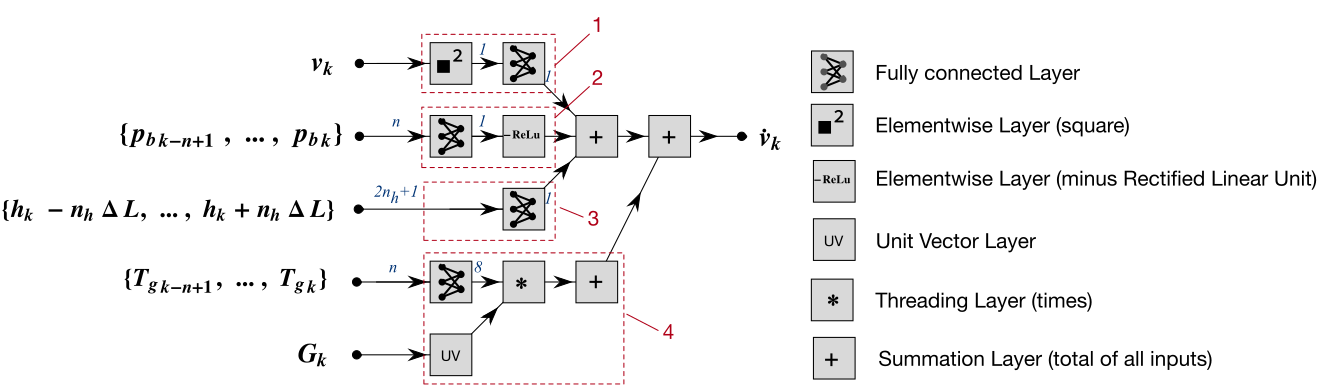• Deal with uncertainties via reinforcement lerning:
• Simulation for Action biasing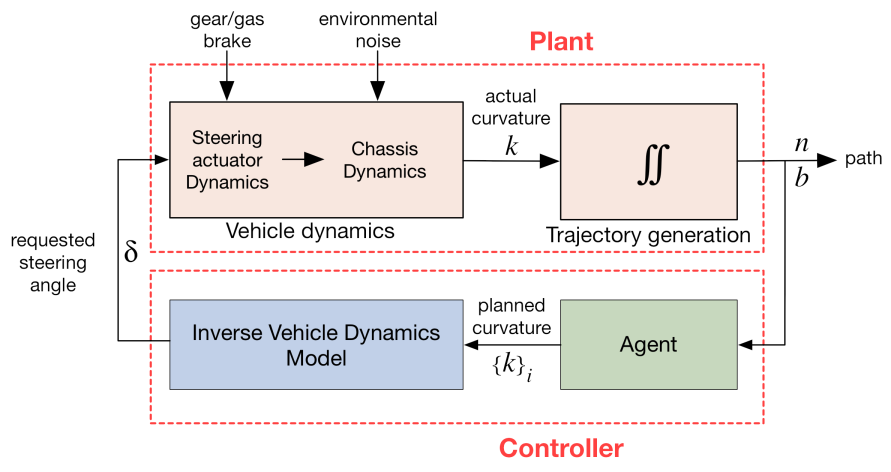— Da Lio, M., Riccardo, D. and Rosati Papini, G.P. "Agent Architecture for Adaptive Behaviours in Autonomous Driving" working progrss...

— Donà, R., Rosati Papini, G.P. et al. "On the Stability and Robustness of Hierarchical Vehicle Lateral Control With Inverse/Forward Dynamics Quasi-Cancellation." IEEE Transactions on Vehicular Technology, 2019.

— Donà, R., Rosati Papini, G.P. et al. "MSPRT action selection model for bio-inspired autonomous driving and intention prediction" 2019 IROS workshop.

— Da Lio, M., Bortoluzzi, D. and Rosati Papini, G.P. "Modelling longitudinal vehicle dynamics with neural networks." Vehicle System Dynamics, 2019.

Rosati Papini, G.P. et al. "A Reinforcement Learning Approach for Enacting Cautious Behaviours in Automated Driving Agents: Safe Speed Choice in the Interaction with Distracted Pedestrians." working progrss...

# Modelling longitudinal vehicle dynamics with neural networks

Causal system\definecolor{myRed}{RGB}{234,26,8} \definecolor{myGreen}{RGB}{50,153,5} \definecolor{myBlue}{RGB}{0,10,206} \definecolor{myYellow}{RGB}{205,255,3} \begin{aligned} {\color{myYellow}a} &= \frac{1}{M} \sum_{i=1}^{m} F_{i} \\ &= {\color{myRed}F_\text{b}}+{\color{myGreen}F_e g_r} + {\color{myBlue}F_a} + {\color{white}F_s} \end{aligned}
Flexible vehicle model with NN

# Network models for longitudinal vehicle dynamics

Neural networks with inspired physical structure
ConvolutionalRecurrent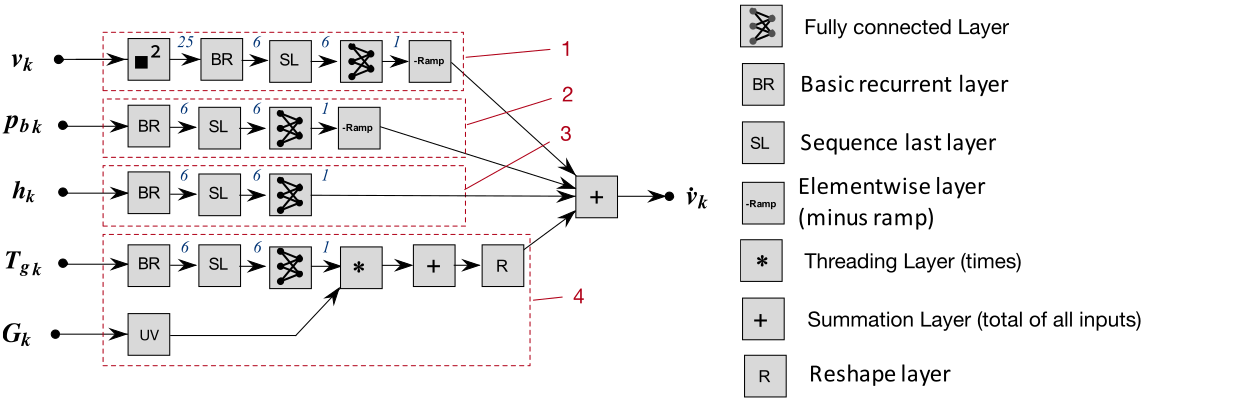Unstructured networks
Convolutional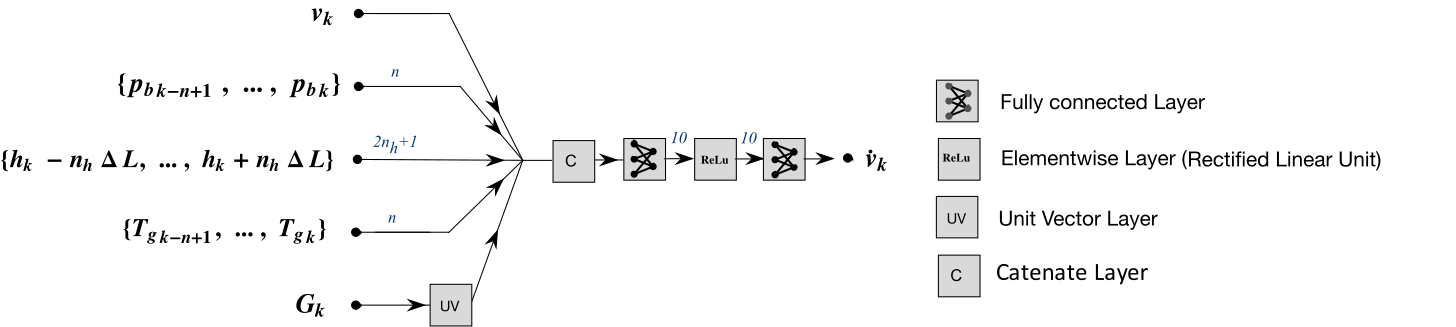Recurrent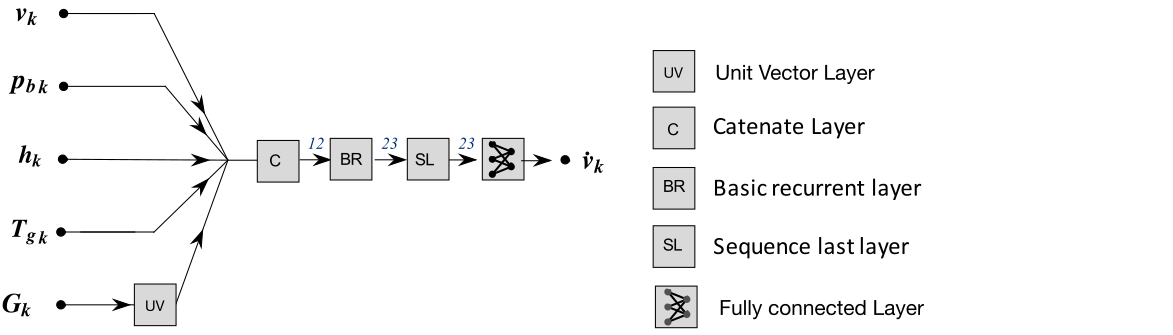All network models

# Network Predictions - time series analysis— Da Lio, M., Bortoluzzi, D. and Rosati Papini, G.P. "Modelling longitudinal vehicle dynamics with neural networks." Vehicle System Dynamics, 2019.
Network Training

# Physical inspiration for data driven approach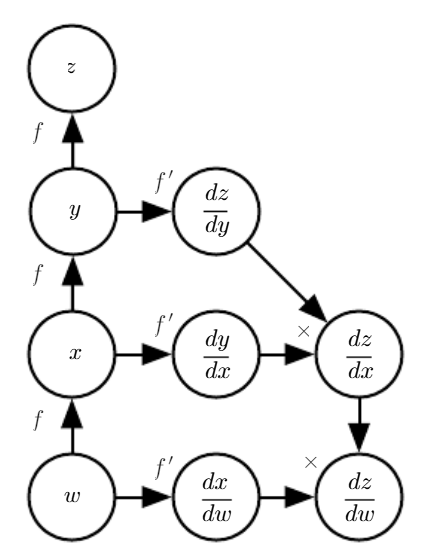The model of the system is realized by neural network that is a:
• differentiable computational graph
• gradient descent algorithms are applicable
In this framework is simple to learn parameters of the model

In our case the characteristics are:
• this approach is flexible and modular
• the structure of the neural network takes inspiration from equations of motion
• the network is no black-box but it is explainable
• less parameters, less data for training, no overfitting, less experiments

— Goodfellow I., Bengio Y. and Courville A. Deep learning. 2015.

# Use neural network for control

Synthesis of neural network for inverse modelling
using direct and inverse neural network in series (unsupervised method)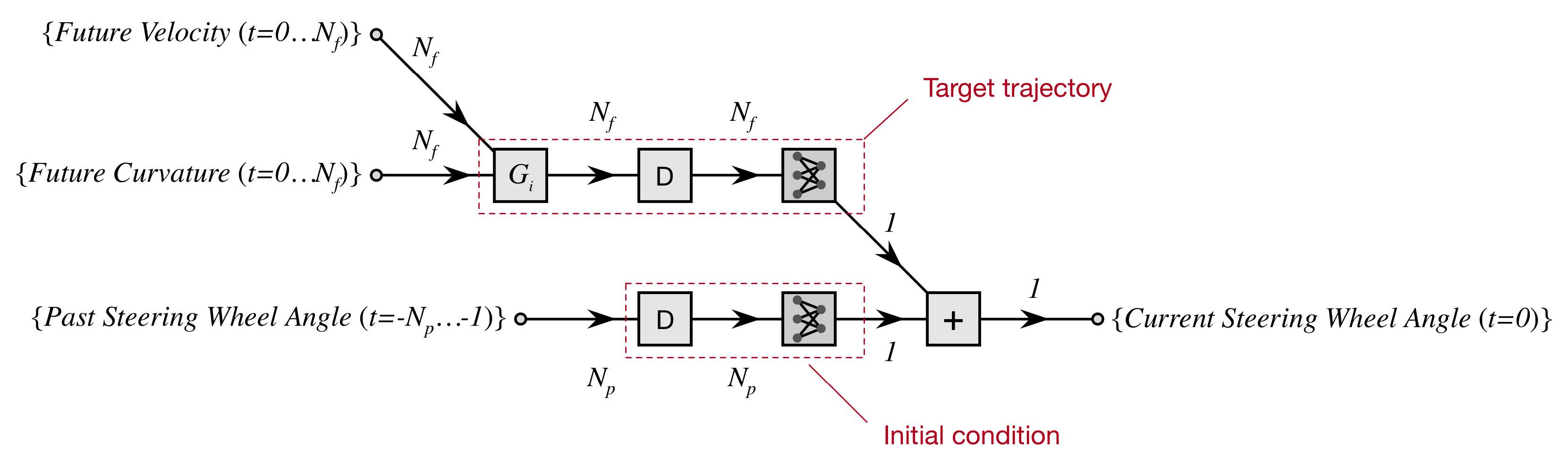Neural networks for control

Miacar (DKFI)
CarMaker
OpenDs

# Deal with uncertain situation

A pedestrian walks on the sidewalk and suddenly may cross the road
Training scenario

# A Reinforcement learning for high-level behaviours

A Reinforcement learning framework integrated autonomous agent:
• I learn only a single baiasing parameter using a neural network, the safe speed
• Simple network to reduce the learning time, and to increse the interpretability
Deal with uncertain situation via RL

# Neural Network for RLThe neural network chooses the requested cruising speed:
• the network possible actions are $$a=\{-\Delta_\text{RCS},0,\Delta_\text{RCS}\}$$
• the network estimates future reward for each possible actions:
• $$Q(s,a)\approx E\left[\sum_k^{\infty} \gamma^{k} r_{t+k}| s, a\right]$$
• a positive reward is given when the car reach without impact the end of the road
• during the simulation data are collected to train the network to a better estimation
Neural network
for RL

# Results - CRF Test of transfer learning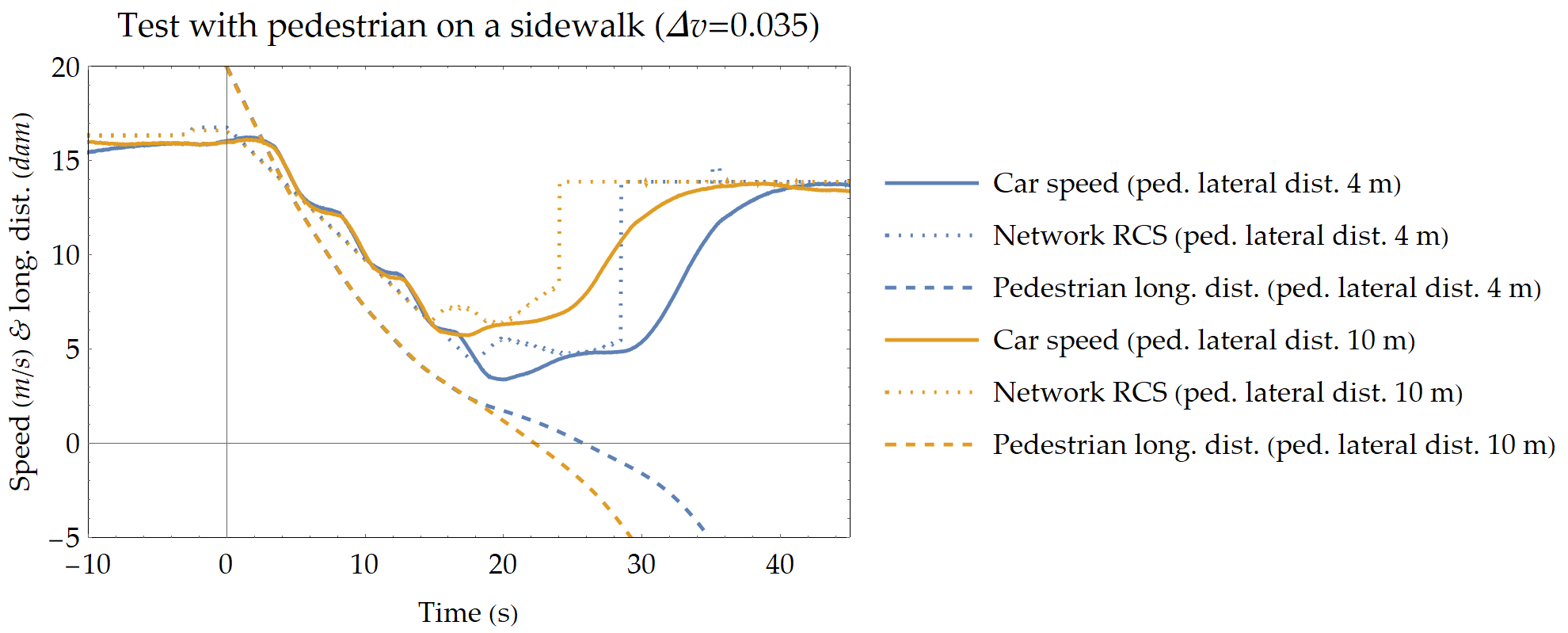# Explainable RL approach on a focused issueDeep Q-learning a reinforcement learning method
• temporal difference
• off policy
• value-based
• model-free

In our case the characteristics are:
• the network used is simple and therefore explainable
• use reinforcement learning only to learn what it is needed
• the network is integrated with an autonomous agent
• the transfer learning is applicable
• it is possible to synthesize a simple logic from the NN behavior

# Future Research

Synergetic collaborations at University of Trento and beyond

# Future of my reseach - Methodological aspects

The concept is not to forget what we know! → but appreciating
• Years of mechanical models
• Established control theory
• Modularity of neural network with physical structure
• Powerfulness of reinforcement learning for high-level behaviors

Neural network open topics:
• Hot swapping of inverse models
• Neural network on-line training
• Observer with auto-tuning
• Robust inverse control
RL open topics:
• Structured network in Q-Learning
• Transfer learning
• Similarities with Optimal Control

# Future of my reseach - Applications

The idea is to apply the techniques explored so far to other fields of research
Future

# Robots - Quadruped

Collaboration: Andrea Del Prete researcher Dep. Industrial Engineering UniTN
HyQ Blue iit robot granted to DII UniTn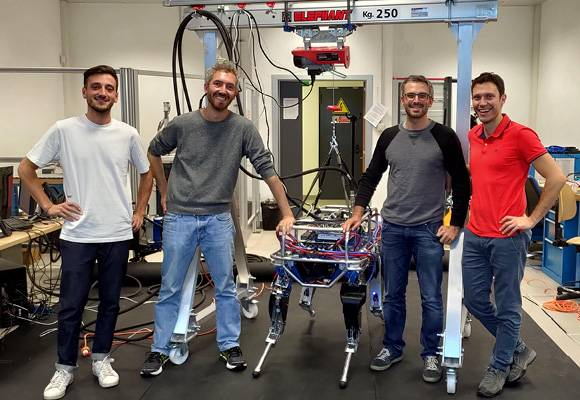Challenges/Opportunities:
• Estimation of perturbation forces
• Estimation of contact forces
• Handling structured objects (doors, drawers, etc..)
• RL application high-level behaviours
• Extension of the notion of motor cortex as way for motor control planning
Robots

Vehicles

# Vehicles - Driving simulator

Collaboration: Prof. Mauro Da Lio Dep. Industrial Engineering UniTN
Challenges/Opportunities:
• Integrate the autonomous agent with the simulator
• Use autonomous agent for realistic traffic
• Studing the human robot interaction, where the human influeces the behavior of the autonomous agent
DII driving simulator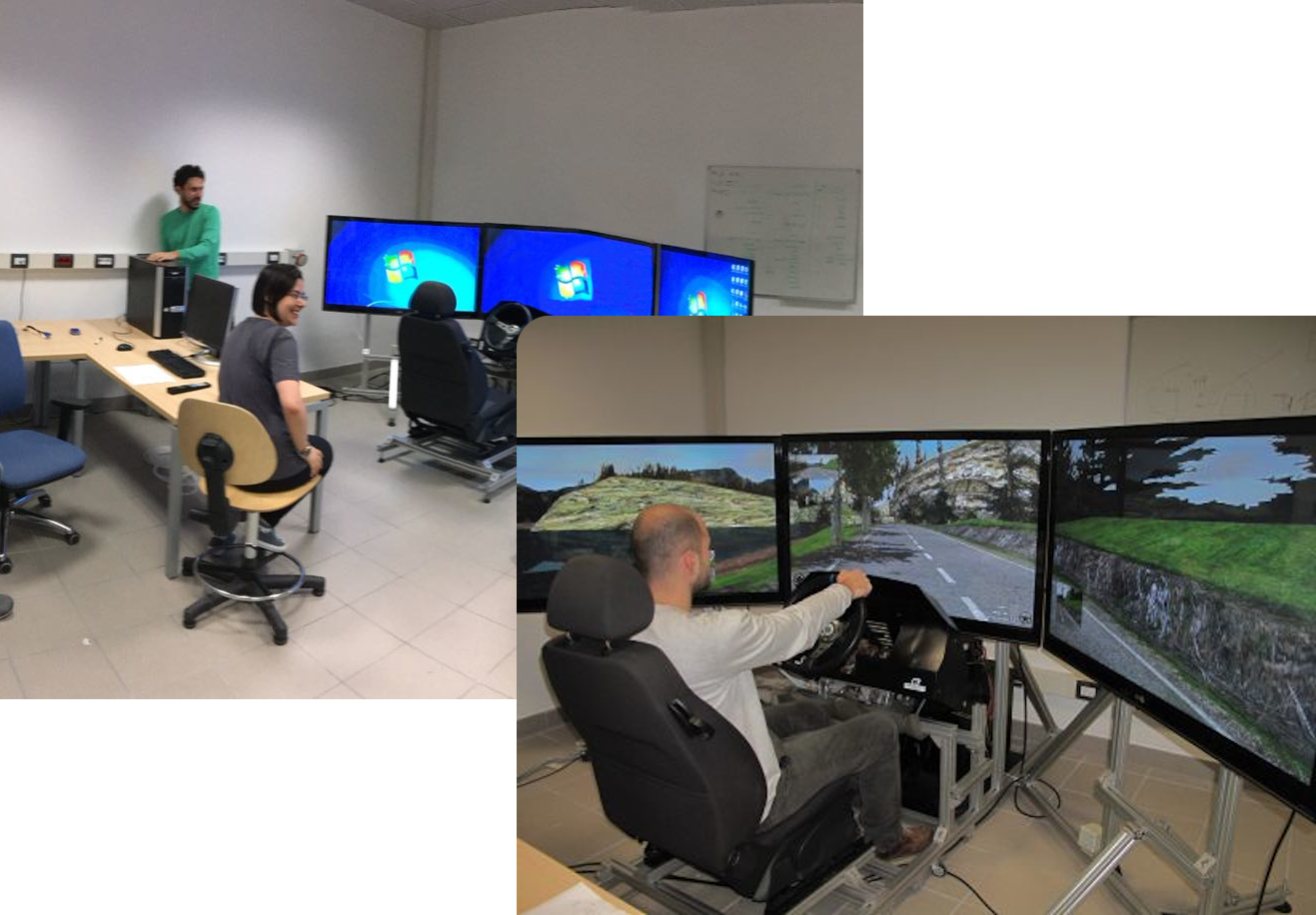Simulator

# Vehicles - Real vehicles

Collaboration: Prof. Paolo Bosetti Dep. Industrial Engineering UniTN
DII Formula SAE Project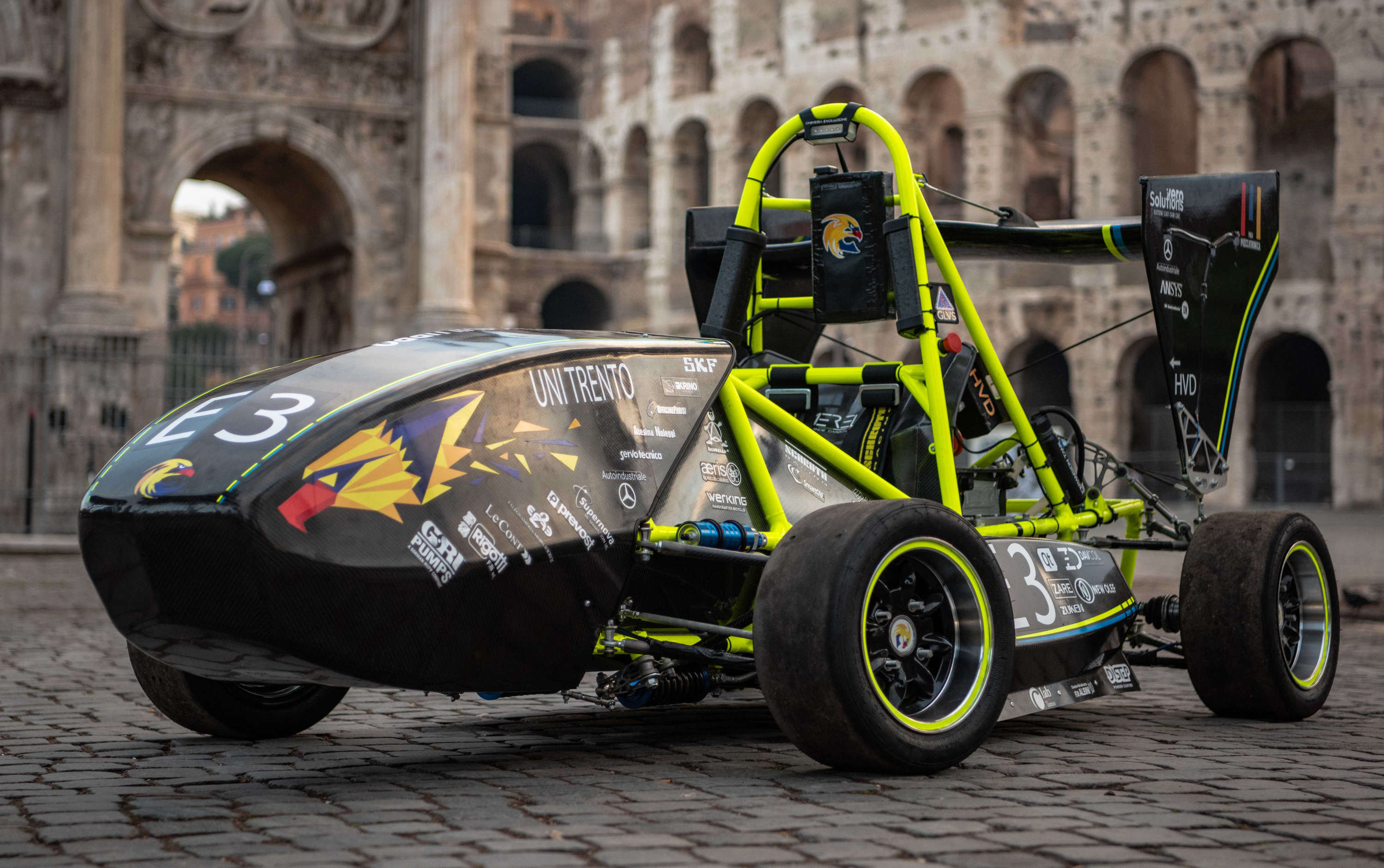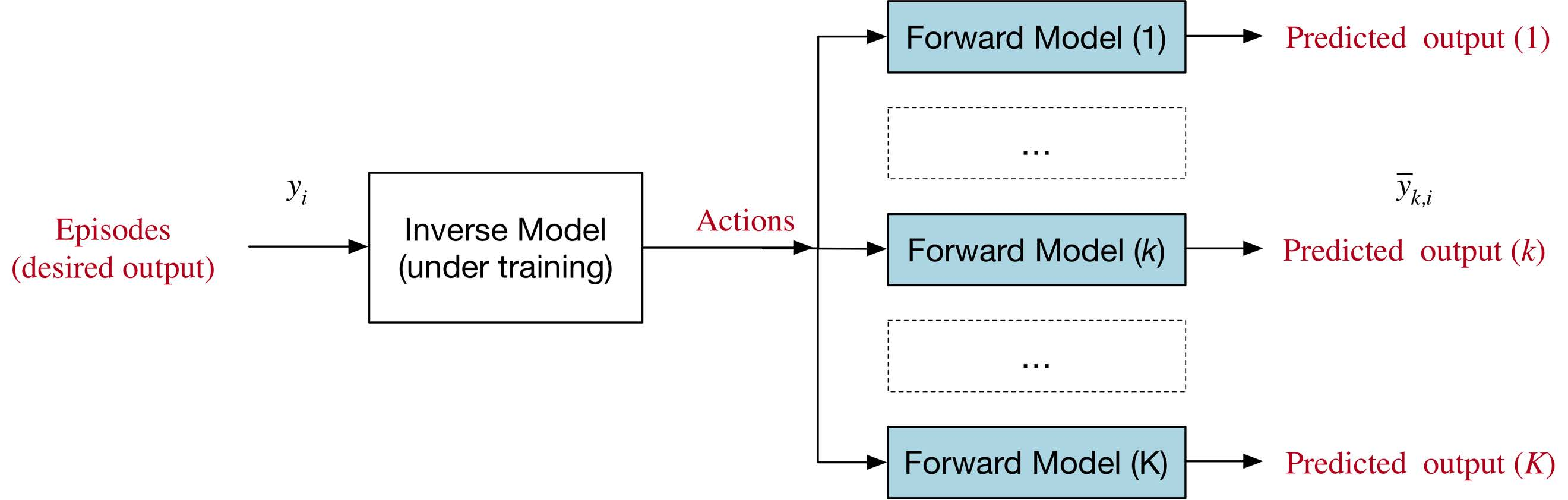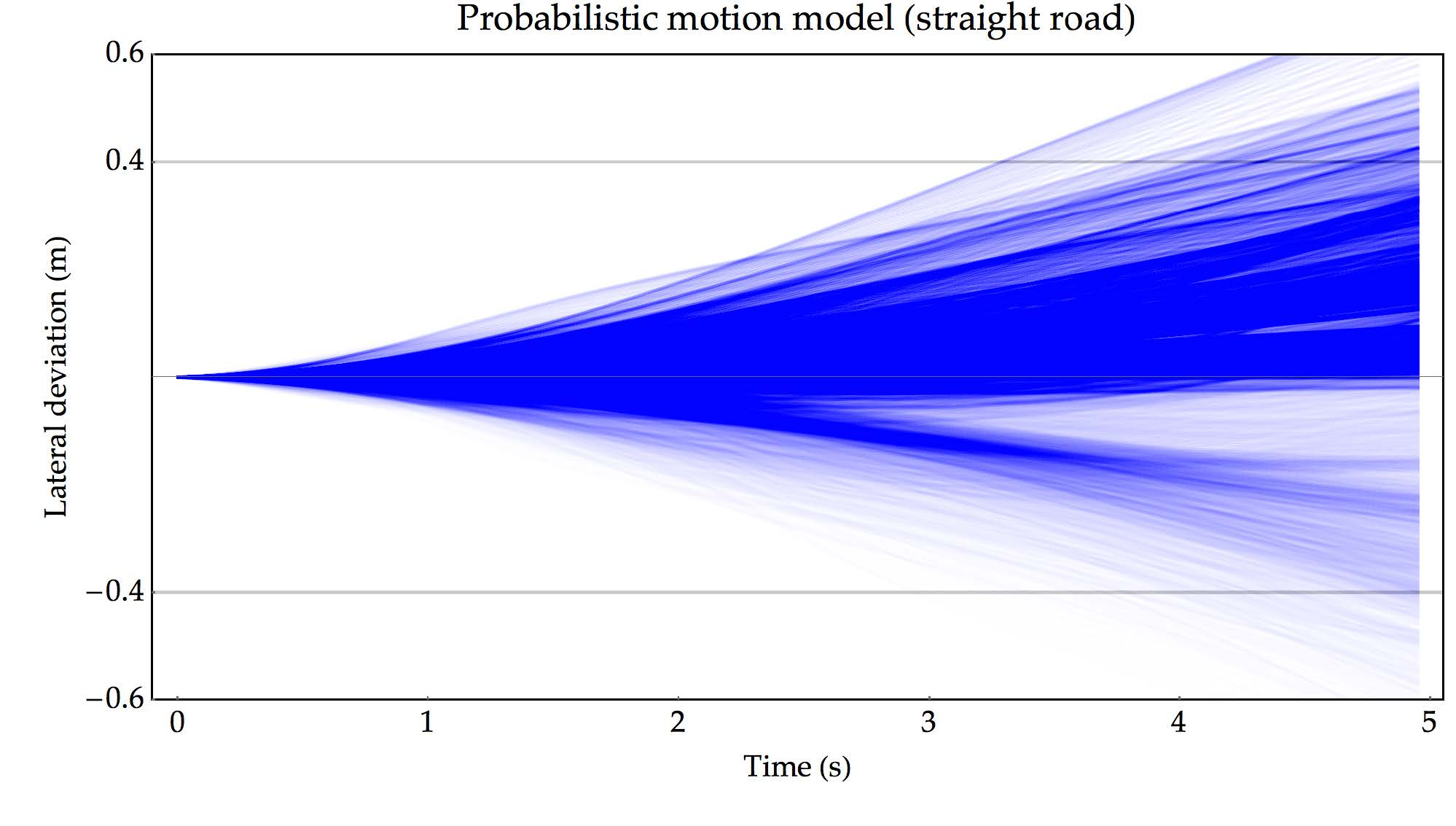Challenges/Opportunities:
• Integrate the autonomous angent with the driving system of the car
• Develop a inverse network for driving to the limits
• Test robust network in real application
Real vehicles

# Smart materials - Solve modelling

Collaboration: Prof. Marco Fontana Dep. Industrial Engineering UniTN
Use physical inspiration to build a neural network model of electroactive polymers$$f_\text{tot} = f_\text{e}(\lambda)+ f_\text{d}\left(\left[\lambda_t,\ldots,\lambda\right],\left[\dot\lambda_t,\ldots,\dot\lambda\right]\right) + f_\text{v}(V,\lambda)$$
• total force realized: $$f_\text{tot}$$
• streach: $$\lambda$$
• voltage: $$V$$
• elastic force: $$f_\text{e}$$
• viscoelastic and isteretic force: $$f_\text{d}$$
• electric force: $$f_\text{v}$$
Challenges/Opportunities:
• Estimation of streach-force response over time
• Estimation of force-streach response
• Optimization of the test experiments
Smart materials

# Other works - Predictions of human motion by structured neural network

Collaboration: Prof. Daniele Fontanelli Dep. Information Eng. and Computer Science UniTn
The network is based on social force model
• $$\mathbf{f}(t)=m \frac{v^{d}(t) \mathbf{e}^{d}(t)-\mathbf{v}(t)}{\tau}+\sum_{w} \mathbf{f}_{w}^{W}(t)$$• forces on pedestrian: $$\mathbf{f}$$
• pedestrian mass: $$m$$
• pedestrian speed: $$\mathbf{v}$$
• desired velocity: $$v^{d}$$
• waypoint direction: $$\mathbf{e}^{d}$$
• velocity chage rate: $$\tau$$
• obstacle forces: $$\mathbf{f}_{w}^{W}$$
Challenges/Opportunities:
• pass to headed social force model
• estimation of more waypoints
• include pedestrian interaction

— Antonucci, A., Rosati Papini, G.P., Palopoli, L., Fontanelli D. "Generating Reliable and Efficient Predictions of Human Motion: A Promising Encounter between Physics and Neural Networks" 2020 IROS, submitted.
Other works

# Future of my reseach - Funding and international collaborations

Previusly won grants
• Starting Grant Young Researchers UniTN 2019
• Title: Deep-learning framework for modelling and control of mechanical systems
• Founding: 14,635 €
• Wave Energy Scotland - Control programme
• Title: Control of Dielectric Elastomer Generator PTO
• Role: Principal investigator and coordinator on behalf of Cheros s.r.l.
• Founding: 47,000 £
Possible grant to apply
• ERC starting Grant
Horizon Europe framework
International contacts
• Prof. David Forehand
School of Engineering, University of Edinburgh
• Prof. David Windridge
Computer Science, Middlesex University London
• Prof. Rocco Vertechy
Department of Engineering, University of Bologna
• Dr. Elmar Berghöfer
Research Center for Artificial Intelligence
• Prof. Sean R. Anderson
Dept. of Automatic Control, University of Sheffield
• Prof. Henrik Svensson
School of Informatics, University of Skövde
• Prof. Giuseppe Lipari
Department of Informatics, Université de Lille
Funding & More
Thank you!

Gastone Pietro Rosati Papini